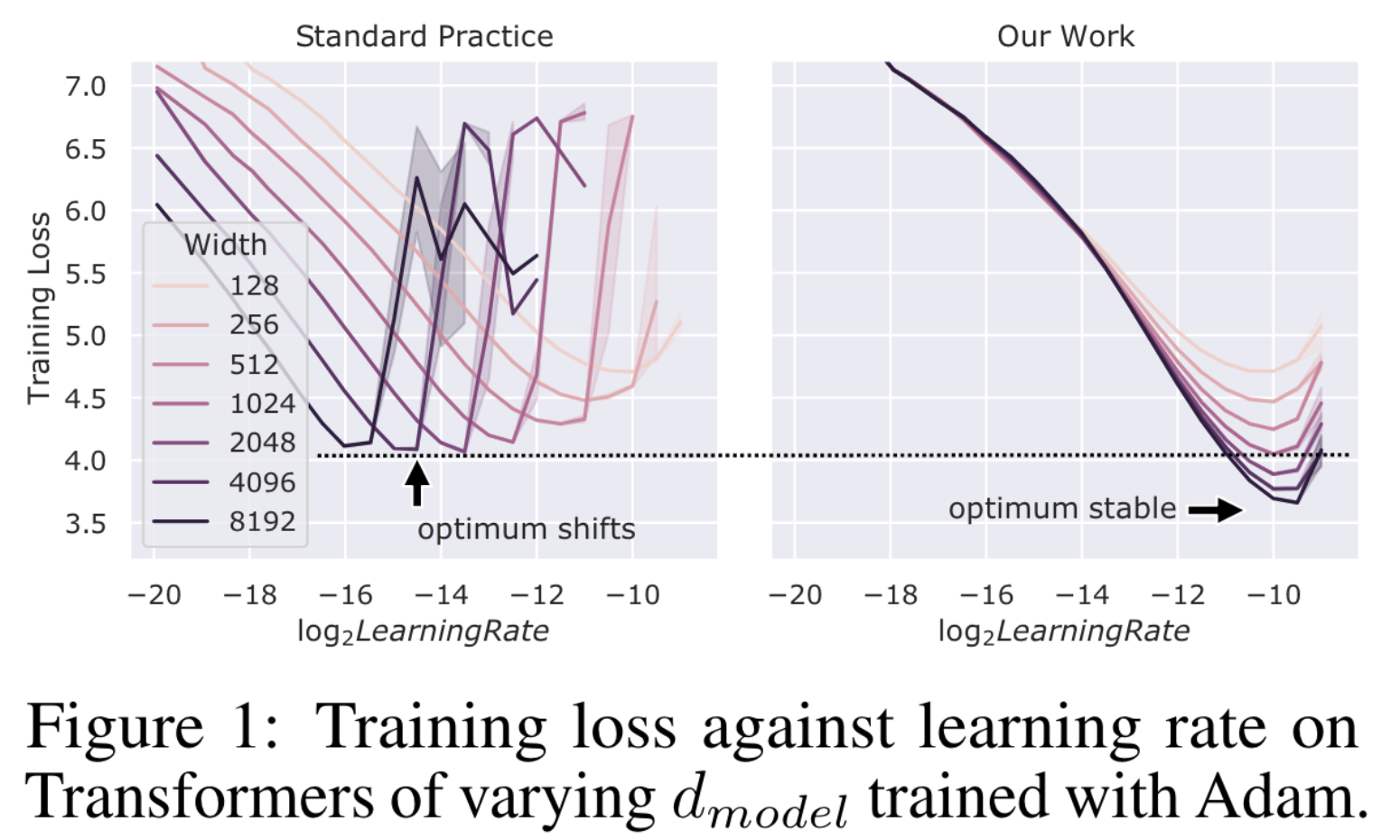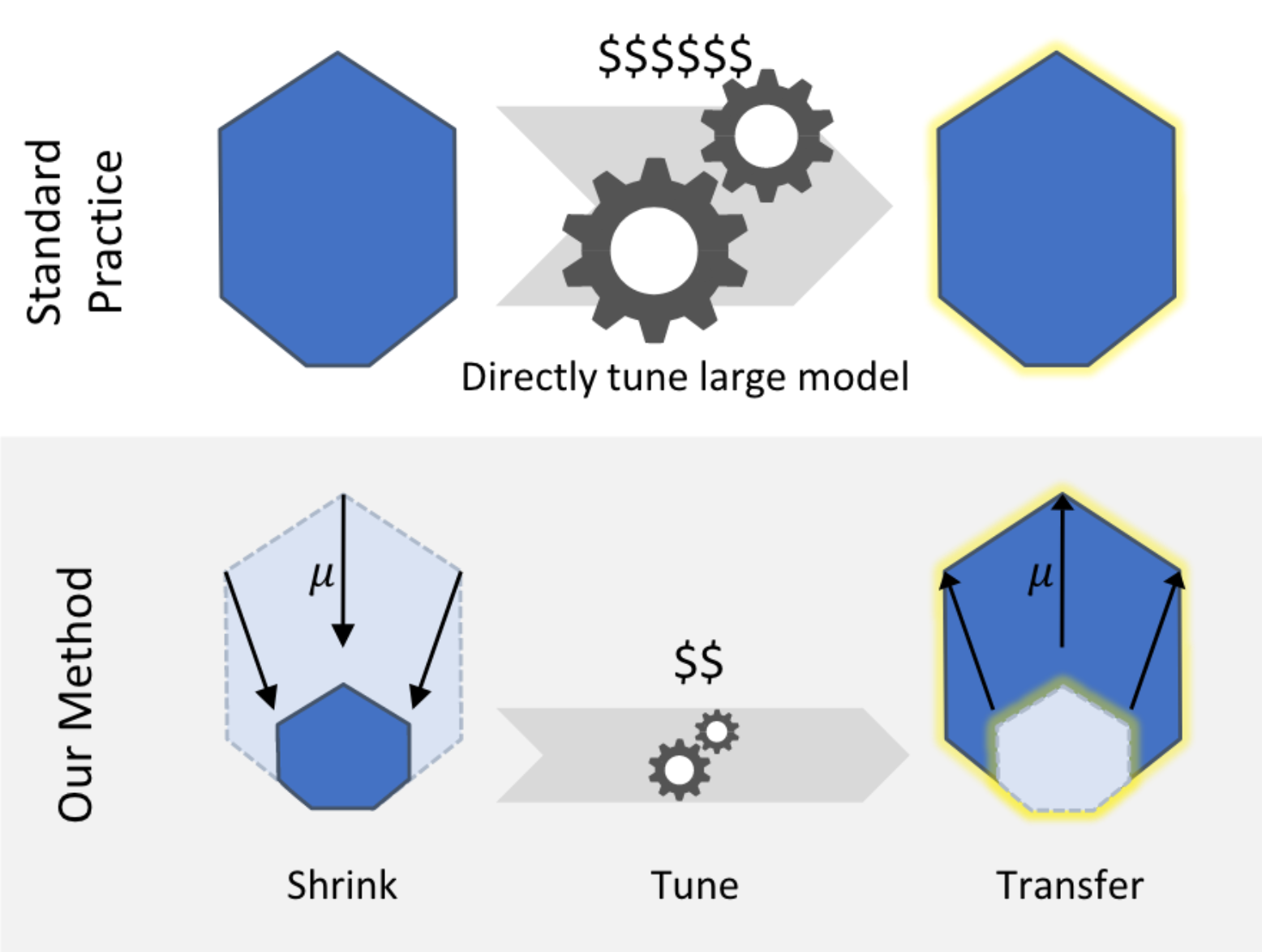# Greg Yang

I am a mathematician at xAI. Previously I was a researcher at Microsoft Research.

I am currently developing a framework called Tensor Programs for understanding large neural networks. This is the theoretical foundation that gave rise to the hyperparameter transfer paradigm for tuning enormous neural networks like GPT-3. Ultimately, my goal is a Theory of Everything for large scale deep learning that 1) tells us the optimal way of scaling neural networks and 2) provides robust understanding to such models so as to guide safety and alignment efforts.

## Tensor Programs

### What is a Tensor Program (TP)?

Informally, a TP is just a composition of matrix multiplication and coordinatewise nonlinearities.

### Why is it important?

#### Universality

It turns out that practically any computation in Deep Learning can be expressed as a TP, e.g. training a transformer on wikipedia data. Simultaneously, any Tensor Program has an “infinite-width” limit which can be derived from the program itself (through what’s called the Master Theorem). So this gives a universal way of taking the infinite-width limit of any deep learning computation, e.g. training an infinite-width transformer on wikipedia data.

#### Theoretical Unification

Theoretically, this generalizes and unifies several lines of previous research, including the Neural Network-Gaussian Process Correspondence, Neural Tangent Kernel, mean field limit of shallow networks, and neural signal propagation, as well as areas outside of deep learning such as random matrix theory and approximate message passing. It also yields the maximal update parametrization and the feature learning limit (see below) for any neural architecture, which would have been hard to derive otherwise without the universality provided by the Tensor Programs machinery.

#### Practical Impact

Practically, this maximal update parametrization (muP), for the first time, allows one to tune the hyperparameters of extremely large neural networks, too expensive to train more than once. This is because, in muP, narrow and wide neural networks share the same set of optimal hyperparameters. So one can tune the large model by just tuning a tiny version of it and copying over the hyperparameters.

How is this different from Neural Tangent Kernel (NTK)? As mentioned above, NTK is a special case of TP. More importantly, a neural network in the NTK limit does not learn features — which contradicts the conventional wisdom that neural networks are successful because of its feature learning capability (e.g. conv nets and BERT). On the other hand, using TP, one can derive a limit (the muP mentioned above) that maximally learns features in a suitable sense (see TP4). When explicitly trained on tasks that depend crucially on feature learning (e.g. Word2Vec), this limit handily beats the NTK limit, as one would expect.

## The Tensor Programs Series

The theory of Tensor Programs is developed (and continues to be developed) over a series of papers.

Scaling Limits of Wide Neural Networks

Arxiv

This is the original paper on Tensor Programs (so it could be named “Tensor Programs O”). It first established the paradigm of 1) using a formal language (Tensor Programs) to capture possible neural computations and 2) deriving an algorithm (the Master Theorem) for taking the infinite-width limit of any such program. All later papers will follow this paradigm.

Unfortunately for readers, the writing was very dense and notation cumbersome, so the next 3 papers focus more pedagogy and presentation of the material to a wider audience, as well as some minor but useful improvements of the results in this paper.

TP1: Wide Neural Networks of Any Architecture are Gaussian Processes

This paper shows that an infinite-width neural network of any architecture (in the standard parametrization) are Gaussian processes at initialization. It also demonstrates how to calculate the kernel of this Gaussian process.

This is a consequence of the simplest form (called Netsor) of Tensor Programs, where one cannot use a matrix and its transpose in the same program, and its Master Theorem. Their theory is fully developed in a self-contained way here.

TP2: Neural Tangent Kernel for Any Architecture

This paper shows that the Neural Tangent Kernel of a neural network of any architecture (in the NTK parametrization) converges to a well-defined, deterministic kernel at initialization. It also demonstrates how to calculate the kernel. (Note it doesn’t yet say anything about training, which is done in Tensor Programs IIb briefed below).

This is a consequence of an extension of Netsor (called NetsorT), where one can use a matrix and its transpose in the same program in a restricted way. Its theory is fully developed in a self-contained way here.

TP2b: Architectural Universality of Neural Tangent Kernel Training Dynamics

Arxiv

This paper continues from the above and shows that 1) the infinite-width Neural Tangent Kernel of a neural network of any architecture (in the NTK parametrization) stays frozen over the course of training, and 2) the infinite-width network evolves via kernel gradient descent with this kernel.

This paper actually needs the machinery of (and comes after) Tensor Programs III (described below), but we number this IIb because the topic is on NTK.

TP3: Neural Matrix Laws

Arxiv

This paper targets a slightly more mathematical audience than the previous papers. It shows how to calculate the Jacobian singular value distribution of an infinite-width neural network. As special cases, it recovers the classical semicircle and Marchenko-Pastur laws.

This is a consequence of the full version of Tensor Programs, where one can use a matrix and its transpose arbitrarily. Its theory is fully developed in a self-contained way here.

This paper is intended to serve as a rigorous reference for the TP foundation going forward.

TP4: Feature Learning in Infinite-Width Neural Networks

This paper derives “the” feature learning limit of neural networks, in contrast to the Neural Tangent Kernel limit, using machinery developed in the previous papers (especially TP3).

(Some trivia: this paper was supposed to be written right after TP0, but I realized the TP foundation needed to be solidified in terms of presentation and notation, hence TP1-3 and why this paper came almost two years later)

TP4b: Adaptive Optimization in the Infinite-Width Limit

This paper classifies all infinite-width limits of general optimization algorithms including Adam and SGD with momentum, strictly generalizing TP4 (which was only about SGD).

To do so, some fundamental advances had to be made: 1) extending TP to a new instruction that can express how adaptive optimizers process gradients into update; 2) borrowing bra-ket notation from quantum physics to express key concepts and calculations in TP much more efficiently.

These advances in the TP machinery makes this the new foundation for papers going forward, subsuming TP3.

A Spectral Condition for Feature Learning

Show that μP is equivalent to scaling the spectral (aka operator) norm of weight matrices like sqrt(fanout/fanin).

A very accessible and pedagogical intro to the scaling (with width) of feature learning. Since the TP series has a reputation for being hard-to-read, this one is not numbered so people will be more likely to read it ;)

TP5: Tuning Large Neural Networks via Zero-Shot Hyperparameter Transfer

You can’t train GPT-3 on a single GPU, much less tune its hyperparameters (HPs).

But what if I tell you…

…you *can* tune its HPs on a single GPU thanks to the theory developed in TP4?

Essentially, narrow and wide neural networks share the same set of optimal hyperparameters if they are in the maximal update parametrization (muP) derived in TP4 (but not if they are in pytorch default parametrization).This lets us transfer hyperparameters from small networks to large networks in the obvious way.The twitter thread above gives a nice but still brief overview of the key ideas and results.

(Some trivia: We finished most of the basic experiments in TP5 before I started writing TP2, at which my motivation for finish writing TP2-TP4 was just to provide enough foundation to write this paper)

TP6: Feature Learning in Infinite-Depth Neural Networks

We extend the results of TP4 and TP5 to infinite-width-and-depth residual networks (resnet).

When the resnet has 1 layer in each block, we can identify a unique optimal parametrization we call Depth-muP, which admits depthwise hyperparameter transfer. In the process, we identify feature diversity as a crucial factor in deep resnets. For example, one can increase feature diversity by switching from relu to absolute value nonlinearity (or anything even would work), and this would greatly increase performance.However, when the resnet has 2 layers in each block, then we find fundamental limitations in all possible infinite-depth limits of such parametrizations.